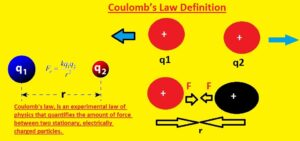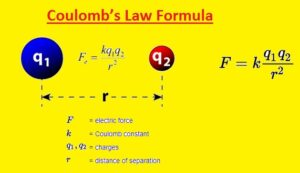Hi, Guys I hope you all are fine and will be doing some fun in your daily life. Friends in today’s tutorial I am going to explain coulomb’s law. This law was first described by a French physicist in 1785, whose name was Charles-Augustin de coulomb’s law. This law is applicable to stationary charges. This law states that the force of attraction and repulsion between two charged particles is directly proportional to the product of the magnitude of charges AND inversely proportional to the square of the distance of charges between them. F12=F21=Kq1q2/r2.
Columb law is applicable to measure force between charged particles. This law is a very important law in physics, which helps us to solve different numerical problems and tells us about stationery charges. In this tutorial, I will explain its detailed description, solved equation, its application, limitations, etc. So, let’s get started with Introduction to Columbs law.

### Introduction to coulomb’s law

•  Coulomb’s law is a law of physic that explain that force between two point charge is directly proportional to their magnitude product and inversely proportional to the squre of the distance between them.
• Its mathematical expression is F x q1q2/r2, F= kq1q2/r2. In this equation F denotes force which is between two charges, q1 is the first charge q2 is the second charge, r is the distance between these two charges and K is proportional.
• In this equation, K is constant its values depend on the medium between two charges.
• If there is no specific medium in between two charges then there is free space is the medium, in this case, the values of k is
K=1/4piE0 In this equation E0 is an electrical constant, which is known as the permittivity of free space.
• In si units its value is 8.8 after putting this value in the given eq we get the  Coulomb’s law force in free space is F=q1q2/r
Columb force is a mutual force it means that if q1 exerts a force of q2 then q2 also exerts force on q1.### Explanation of  Coulomb’s law

• As we already know the force between two charges is mutual force, if we denote force exerted by on q2 by q1 is F21 and on q1 by q2 F12 then we have an equation like this
• F12=-F21 in this equation minus sign shows that the direction of these forces is opposite.
• If r21 is a unit vector that has a direction from q1 to q2 and r12 has a direction from q2 to q1 then we have an equation like this
Both forces are shown in given diagram.• Now, let we consider if there is a medium between these two charges then what will happen?
If the medium is an insulator it is also known as a dielectric. It is observed that when there is a medium between two charge particles then the force of attraction becomes less by certain factors. The decrease in values of this force depends on the medium because every medium has its certain values for the decrease in force.

### Coulomb’s Law Definition

• Coulomb law states that interaction between charged particles. That defines the number of electrostatic forces existing between eh two charged objects that is repulsion and repulsion
• If the charges are the same they will have the force of repulsion and opposite charges will have the force of attraction### Coulomb’s Law Formula

• In the above figure, you can see the formula of the coulomb law. This expression shows that the force existing between two charged particles is equal to the product of two charges Q1 and Q2 divided by the square of the distance between charges denoted with r. In this formula K is constant
• The unit used for this force is Newton. Since distance is squared as the distance between two objects is doubled force between them decreases to four times### Coulomb’s Law vs Universal Law of Gravitation

• There is some similarities exist between this two law but they are different
• The electric force is stronger than gravity
• In the below equations, you can see that there are different proportionality constants used for these two forces
• In gravity, mass is used for force calculation, and for coulomb law charge is used to measure the force
• Gravitational force is always attractive and electrostatic force is repulsive or attractive according to charges polarity for same charges will be repulsive and different charges will be attractive### Vector Form of Coulomb’s Law

• As the force of electrostatic is like force so interaction between charges is a vector quantity that direction and magnitude.. In other words values of direction and magnitude of electric force
• If we make some changes to our coulomb law equation then we have• The arrows existing in the equation denote the vector quantity
• This expression is unit vector that has a direction from charge Q1 to charge Q2 and is denoted as 12 which means charge Q1 applies the force on charge Q2
• If we have positive results from the equation we can say that forces are repulsive and for opposite charges or attraction forces minus sign will exist

### Limitations of Coulomb’s Law

• There are some limitation this law have described here
• It is used for static charges not for moving charges
• It is used to find the forces between charges that have a regular shape not used for irregular shapes
• The formula of this law is used only if molecules of solvent among the particles are larger than both charges
• Where inverse square law is applied it used

That is all about the coulomb’s law all details has explained if you have any questions ask here